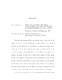## FAST AND OPTIMAL SOLUTION ALGORITHMS FOR PARAMETERIZED PARTIAL DIFFERENTIAL EQUATIONS

 dc.contributor.advisor Elman, Howard C en_US dc.contributor.author Lee, Kookjin en_US dc.contributor.department Computer Science en_US dc.contributor.publisher Digital Repository at the University of Maryland en_US dc.contributor.publisher University of Maryland (College Park, Md.) en_US dc.date.accessioned 2018-01-23T06:44:49Z dc.date.available 2018-01-23T06:44:49Z dc.date.issued 2017 en_US dc.description.abstract This dissertation presents efficient and optimal numerical algorithms for the solution of parameterized partial differential equations (PDEs) in the context of stochastic Galerkin discretization. The stochastic Galerkin method often leads to a large coupled system of algebraic equations, whose solution is computationally expensive to compute using traditional solvers. For efficient computation of such solutions, we present low-rank iterative solvers, which compute low-rank approximations to the solutions of those systems while not losing much accuracy. We first introduce a low-rank iterative solver for linear systems obtained from the stochastic Galerkin discretization of linear elliptic parameterized PDEs. Then we present a low-rank nonlinear iterative solver for efficiently computing approximate solutions of nonlinear parameterized PDEs, the incompressible Navier–Stokes equations. Along with the computational issue, the stochastic Galerkin method suffers from an optimality issue. The method, in general, does not minimize the solution error in any measure. To address this issue, we present an optimal projection method, a least-squares Petrov–Galerkin (LSPG) method. The proposed method is optimal in the sense that it produces the solution that minimizes a weighted l2-norm of the solution error over all solutions in a given finite-dimensional subspace. The method can be adapted to minimize the solution error in different weighted l2-norms by simply choosing a specific weighting function within the least-squares formulation. en_US dc.identifier https://doi.org/10.13016/M2XP6V52R dc.identifier.uri http://hdl.handle.net/1903/20388 dc.language.iso en en_US dc.subject.pqcontrolled Computer science en_US dc.subject.pqcontrolled Applied mathematics en_US dc.title FAST AND OPTIMAL SOLUTION ALGORITHMS FOR PARAMETERIZED PARTIAL DIFFERENTIAL EQUATIONS en_US dc.type Dissertation en_US
##### Original bundle
Now showing 1 - 1 of 1